# What is 10

What is the 5th term, if the 8th term is 80 and common ratio r =1/2?

a5 =  640

### Step-by-step explanation: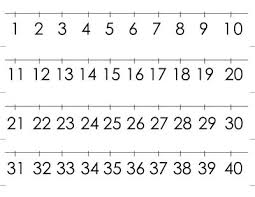Did you find an error or inaccuracy? Feel free to write us. Thank you!## Related math problems and questions:

• If the 3If the 6th term of a GP is 4 and the 10th is 4/81, find common ratio r.
• Geometric progressiobIf the sum of four consective terms of geometric progression is 80 and arithmetic mean of second and fourth term is 30 then find terms?
• Arithmetic progression 2The 3rd term of an Arithmetic progression is ten more than the first term, while the fifth term is 15 more than the second term. Find the sum of the 8th and 15th terms of the Arithmetic progression if the 7th term is seven times the first term.
• Gp - 80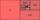One of the first four members of a geometric progression is 80. Find its if we know that the fourth member is nine times greater than the second.
• HP - harmonic progression 2Compute the 16th term of the HP if the 6th and 11th term of the harmonic progression are 10 and 18 respectively.
• Geometric progressionIn geometric progression, a1 = 7, q = 5. Find the condition for n to sum first n members is sn≤217.
• GP membersThe geometric sequence has 10 members. The last two members are 2 and -1. Which member is -1/16?
• Five element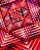The geometric sequence is given by quotient q = 1/2 and the sum of the first six members S6 = 63. Find the fifth element a5.
• Common difference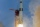The 4th term of an arithmetic progression is 6. If the sum of the 8th and 9th terms is -72, find the common difference.
• 10th termWhat is the 10th term of the Arithmetic Progression if x1=4 and d=5?
• Missing term 2What is the missing term for the Geometric Progression (GP) 3, 15, 75,__, 1875?
• Find the 2Find the term independent of x in the expansion of (4x3+1/2x)8
• HP - harmonic progressionDetermine the 8th term of the harmonic progression 2, 4/3, 1,…
• Quotient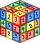Determine the quotient and the second member of the geometric progression where a3=10, a1+a2=-1,6 a1-a2=2,4.
• Sequence 11What is the nth term of this sequence 1, 1/2, 1/3, 1/4, 1/5?
• 15th term of APWhat is the 15th term; x1=1.5, d=4.5?
• Two geometric progressions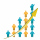Insert several numbers between numbers 6 and 384 so that they form with the given GP numbers and that the following applies: a) the sum of all numbers is 510 And for another GP to apply: b) the sum of entered numbers is -132 (These are two different geome